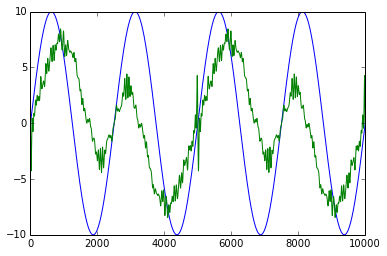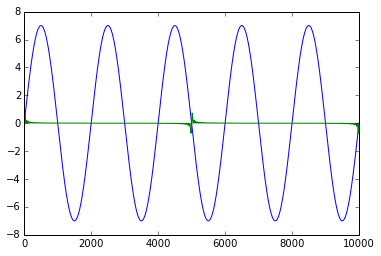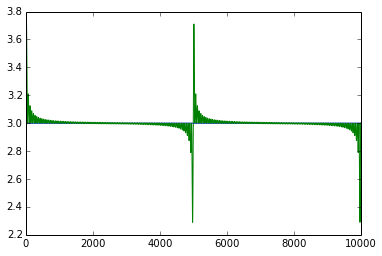## Friday, April 11, 2014

### PCA and ICA 'Learn' Representations of Time-Series

PCA is usually demonstrated in a low-dimensional context. Time-series, however, are high dimensional and ICA might be a more natural technique for reducing dimensionality.

For my current project, I'm not interested in dimensionality reduction per-se; rather I'm interested in how well, given a reduced representation of some base input time-series, how well the algorithm can reproduce a new input. If the new input cannot be recreated well, then it is a candidate for being considered an anomaly.

I've setup an experiment where I generated a bunch of even number sine waves in the domain as (base/training) input to the algorithms plus a constant function. Then I try to reconstruct a slightly different even sine wave, an odd sine wave, and a constant.

The result is that the even sine wave and constant are somewhat reconstructed while the odd sine wave is not. You can see this in the following graphs where the blue line is a 'target' signal and the green line is the reconstructed signal. I get similar results using PCA.
4 waves5 waves FAILconstant = 3There are plenty of mathematical rigor and algorithmic parameters that I haven't talked about but this is a post that requires minimal time and technical knowledge to go through. However, you can figure out details if you examine the ipython notebook.